# Find the Line Count of a File in Linux Bash

Yahya Irmak Mar 22, 2022 Jan 02, 2022

This article will explain how to find the line count of a file in Linux Bash. We will give example usages of `wc`, `grep`, `cat`, `sed`, `awk`, and `nl` tools.

There is more than one way to find the number of lines in Linux. Let’s create our sample file and count its line numbers using different tools.

Below is the content of the file we will use in this example. Save it as example.txt.

``````line1
line2
line3
line4
line5
``````

## Use `wc` to Count Number of Lines in Bash

The `-l` parameter of the `wc` command returns the number of lines.

``````wc -l < example.txt
``````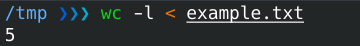## Use `grep` to Count Number of Lines in Bash

The `-c` parameter of the `grep` command returns the number of lines.

``````grep "" -c example.txt
``````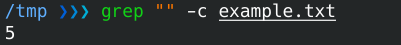## Use `cat` to Count Number of Lines in Bash

The `cat` command prints the file contents to the console in numbered format with the `-n` parameter. We get the last line with the `tail` command, and with `awk`, we get the line number.

``````cat -n example.txt | tail -1 | awk '{print \$1}'
``````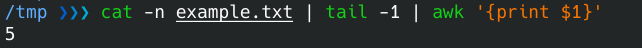## Use `sed` to Count Number of Lines in Bash

We can find the number of file lines with the following use of the `sed` command.

``````sed -n '\$=' example.txt
``````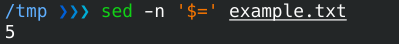## Use `awk` to Count Number of Lines in Bash

The `awk` tool can be used to find the number of file lines.

``````awk 'END{print NR}' example.txt
``````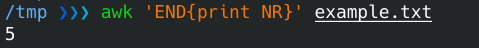## Use `nl` to Count Number of Lines in Bash

The `nl` command prints the file contents to the console in numbered format with the `-n` parameter. We get the last line with the `tail` command, and with `awk`, we get the line number.

``````nl example.txt | tail -1 | awk '{print \$1}'
``````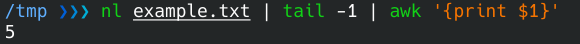Author: Yahya Irmak

Yahya Irmak has experience in full stack technologies such as Java, Spring Boot, JavaScript, CSS, HTML.